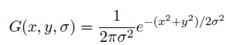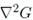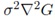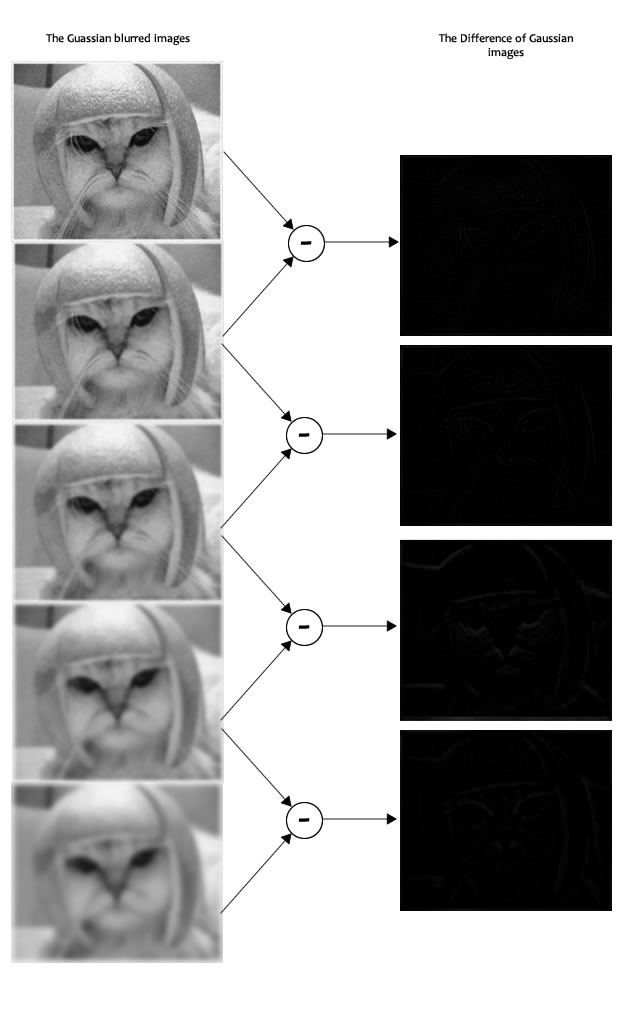## LoG approximations

Series: SIFT: Theory and Practice:

In the previous step , we created the scale space of the image. The idea was to blur an image progressively, shrink it, blur the small image progressively and so on. Now we use those blurred images to generate another set of images, the Difference of Gaussians (DoG). These DoG images are a great for finding out interesting key points in the image.

## Laplacian of Gaussian

The Laplacian of Gaussian (LoG) operation goes like this. You take an image, and blur it a little. And then, you calculate second order derivatives on it (or, the "laplacian"). This locates edges and corners on the image. These edges and corners are good for finding keypoints.

But the second order derivative is extremely sensitive to noise. The blur smoothes it out the noise and stabilizes the second order derivative.

The problem is, calculating all those second order derivatives is computationally intensive. So we cheat a bit.

## The Con

To generate Laplacian of Guassian images quickly, we use the scale space. We calculate the difference between two consecutive scales. Or, the Difference of Gaussians. Here's how:These Difference of Gaussian images are approximately equivalent to the Laplacian of Gaussian. And we've replaced a computationally intensive process with a simple subtraction (fast and efficient). Awesome!

These DoG images comes with another little goodie. These approximations are also "scale invariant". What does that mean?

## The Benefits

Just the Laplacian of Gaussian images aren't great. They are not scale invariant. That is, they depend on the amount of blur you do. This is because of the Gaussian expression. (Don't panic ;) )See the σ2 in the demonimator? That's the scale. If we somehow get rid of it, we'll have true scale independence. So, if the laplacian of a gaussian is represented like this:Then the scale invariant laplacian of gaussian would look like this:But all these complexities are taken care of by the Difference of Gaussian operation. The resultant images after the DoG operation are already multiplied by the σ2. Great eh!

Oh! And it has also been proved that this scale invariant thingy produces much better trackable points! Even better!

## Side effects

You can't have benefits without side effects >.<

You know the DoG result is multiplied with σ2. But it's also multiplied by another number. That number is (k-1). This is the k we discussed in the previous step.

But we'll just be looking for the location of the maximums and minimums in the images. We'll never check the actual values at those locations. So, this additional factor won't be a problem to us. (Even if you multiply throughout by some constant, the maxima and minima stay at the same location)

## Example

Here's a gigantic image to demonstrate how this difference of Gaussians works.In the image, I've done the subtraction for just one octave. The same thing is done for all octaves. This generates DoG images of multiple sizes.

## Summary

Two consecutive images in an octave are picked and one is subtracted from the other. Then the next consecutive pair is taken, and the process repeats. This is done for all octaves. The resulting images are an approximation of scale invariant laplacian of gaussian (which is good for detecting keypoints). There are a few "drawbacks" due to the approximation, but they won't affect the algorithm.

Next, we'll actually find some interesting keypoints. Maxima and Minima. Or, Maximums and Minimums of the image.

## More in the series

This tutorial is part of a series called SIFT: Theory and Practice:

Utkarsh Sinha created AI Shack in 2010 and has since been working on computer vision and related fields. He is currently at Microsoft working on computer vision.